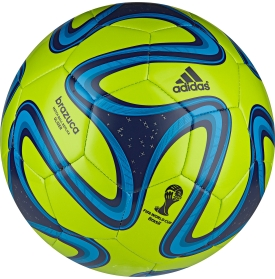# Soccerball Polyhedra Mania

Geometry Level 3

This brazuca soccer ballhas hexagonal faces, pentagonal faces, and some swirls that makes it look covered with crosses, all symmetrically arranged. It seems possible to rotate the ball so that the design realigns with itself (if we disregard printing and logos). The $5$ regular polyhedra are:

I Tetrahedron
II Cube
III Octahedron
IV Dodecahedron
V Icosahedron

A symmetry transformation is a function (or operation) $F(x)$ that leaves $x$ unchanged, that is, $F(x)=x$. If we apply, say, the same symmetry transformation which leaves leaves the tetrahedron unchanged to this soccerball, it will either leave the soccerball unchanged, or not. Which of these solids one can apply all the rotation symmetry transforms to both the regular polyhedron and the soccerball, and leave both unchanged?

Note that only rotation transforms will be considered here, that is, as the soccerball is in play, how can it re-align with itself? Both the seams and the swirls are to re-align with itself.

×